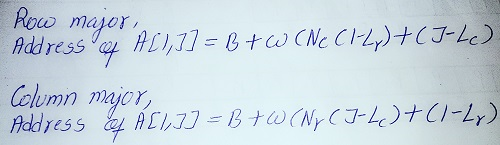Generic selectors
Exact matches only
Search in title
Search in content
Post Type Selectors

# Net 48

CBSE NET
Q. Consider an array A[20, 10], assume 4 words per memory cell and the base address of array A is 100. What is the address of A[11, 5] ? Assume row major storage.
(A) 560
(B) 565
(C) 570
(D) 575
Ans: (A)
Explanation:
Formula for calculating address of element in row major and column major order is as shown in below image.Where,
B=Base address, w=element size, Nc=number of column, Nr=number of row, Lc=column lower bound, Lr=row lower bound.
Using formula of row major,

Address of (11,5) = 100+4(10(11-0)+(5-0))= 100+4(115)=560.